Latest Banking jobs   »

# Quantitative Aptitude Quiz For IBPS SO Prelims 2022- 29th November

Directions (1-5): Study the bar chart given below and answer the following questions.
Bar chart shows the percentage of growth in revenue of company-A & B over 5 years period (2014-2018) with respect to previous year. Revenue of company – A & B at the end of 2013 is Rs.20000 & Rs.50000 respectively.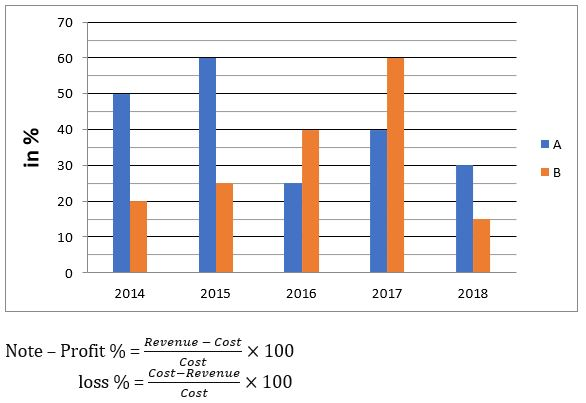Q1. If in 2015 company-A earned 20% profit and company-B incurred loss of 6 ¼ %, then find overall profit or loss earned by company – A & B together in 2015.
(a) Rs.8000
(b) Rs.10000
(c) Rs.3000
(d) Rs.6000
(e) Rs.7000

Q2. Find difference between average revenue of company-A in 2016 & 2017 and average revenue of company-B in 2014 & 2016.
(a) Rs.13000
(b) Rs.6000
(c) Rs.10000
(d) Rs.15000
(e) None of the above.

Q3. Find percentage increase in revenue of company-A in 2018 as compared to revenue of company-A in 2014.
(a) 264%
(b) 252%
(c) 282%
(d) 268%
(e) 276%

Q4. If in 2017 company-B earned a profit of 12% and in 2018 company-A earned a profit of 4%, then find cost of company-A in 2018 is what percent of cost of company-B in 2017?
(a) 80%
(b) 55%
(c) 40%
(d) 70%
(e) 95%

Q5. Find ratio of revenue earned by company-B in 2018 to revenue earned by company-A in 2017.
(a) 18 : 13
(b) 26 : 17
(c) 15 : 4
(d) 23 : 10
(e) None of the above.

Directions (6 – 10): The following table shows the total no. students and percentage of students present on a particular day out of them for two schools A and B in different classes.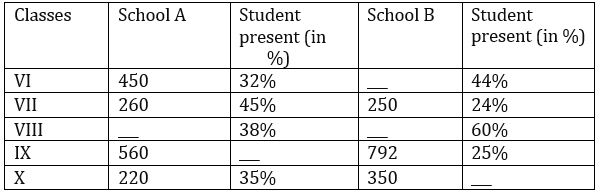Q6. If total number of students in class VIII in both the school together are 1625, while total students present in both the classes together are 766, then find difference between number of students in class VIII in both the schools?
(a)250
(b) 275
(c) 225
(d) 300
(e) None of these

Q7.The number of students present in class VI in school A are approximately what percent of number of students present in class IX in school B?
(a)65%
(b) 70%
(c) 75%
(d) 73%
(e) 80%

Q8. If number of students present in class VI in school B are 2 less than double of the number of students present in same class in school A, then find the total number of students in class VI in school B.
(a)625
(b) 650
(c) 600
(d) 700
(e) None of these

Q9.If no. of students present in class XI in school B are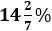less than number of students present in class X in the same school, while total students in class XI in school B is 400, then what percent of students in class XI in school B are present on that day?
(a)25.5%
(b) 26.5%
(c) 27.5%
(e) None of these

Q10. Find the total number of students present in class VII in both the schools together?
(a) 157
(b) 187
(c) 167
(d) 177
(e) None of these

Direction (11–15): The given graph shows the number of students studying in three different classes (Class VI, Class VII & Class VIII) of six different schools. Read the information carefully and answer the following questions.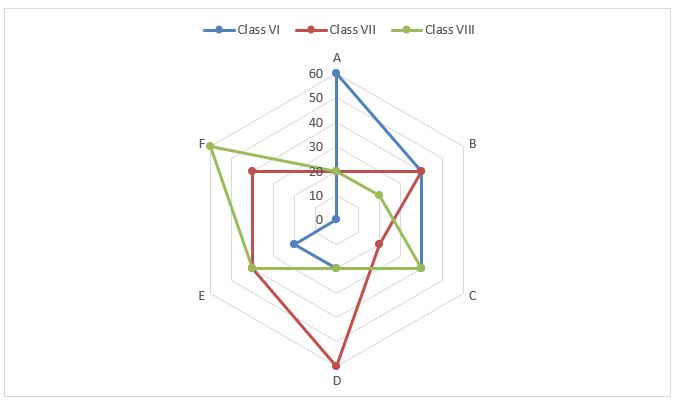Q11. Total number of students in school D in all three classes are what percent of total number of students in all three classes in school F?
(a) 40%
(b) 50%
(c) 60%
(d) 75%
(e) 100%

Q12. Total number of class VI students in school A, B & C together is how much more or less than the total number of class VIII students in school D, E & B together?
(a) 40
(b) 50
(c) 30
(d) 80
(e) 60

Q13. Find the average number of class VII students in all the schools except F.
(a) 36
(b) 32
(c) 30
(d) 38
(e) 40

Q14. Find the ratio of total number of students in school A to total number of class VIII students in school C, D & E together.
(a) 3 : 4
(b) 1 : 1
(c) 2 : 3
(d) 3 : 2
(e) 4 : 3

Q15. Which of the following schools has highest number of students studying in these three classes?
(a) A
(b) B
(c) C
(d) D
(e) All of the above

Solutions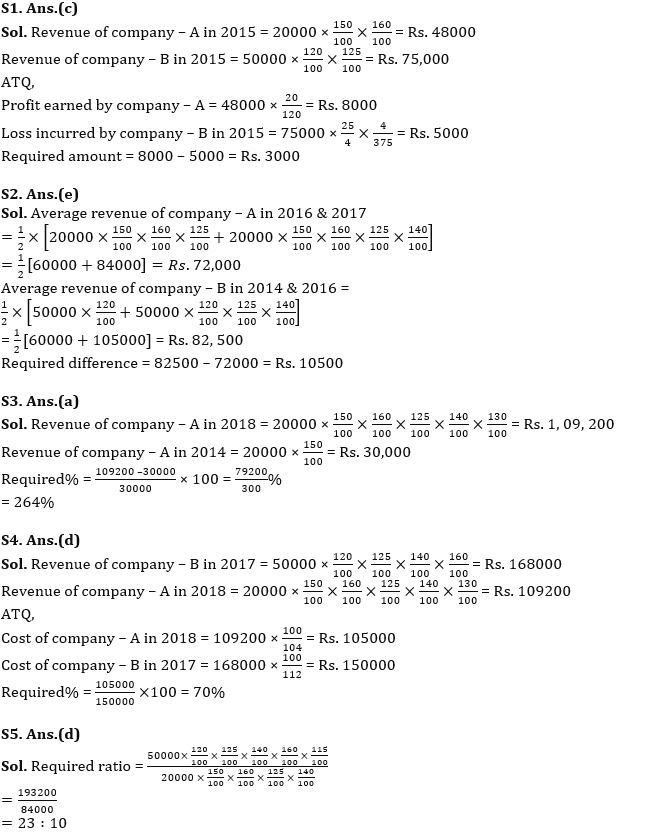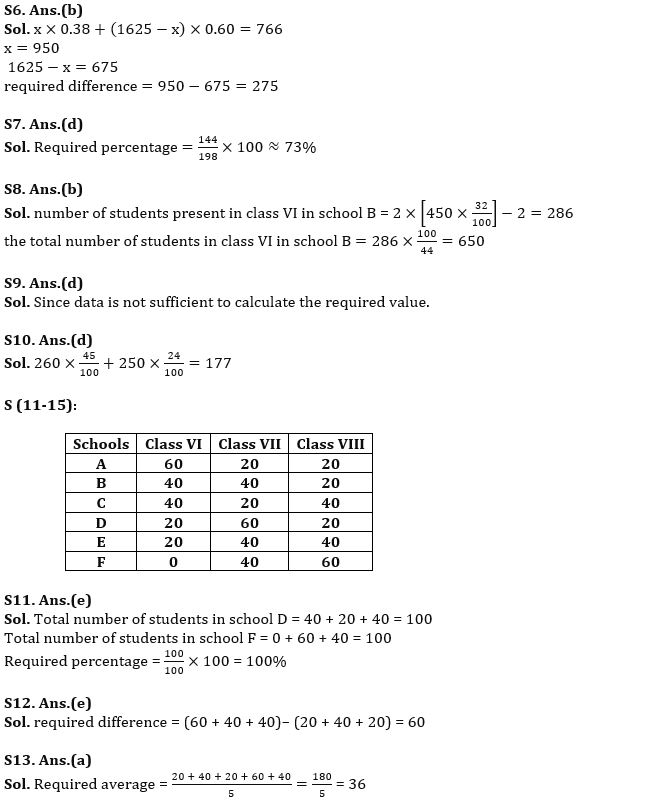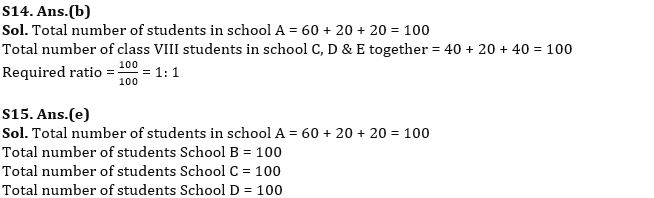#### Congratulations!Union Budget 2023-24: Free PDF